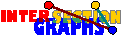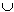# Connection Graphs

Connection graphs are essentially underlying graphs of intersection digraphs. The only difference is that loops of the digraph remain in the connection graph, i.e. connection graphs are undirected graphs with loops allowed. Formally, we have a family (Sx,Tx), xV of pairs of sets. The connection graph has V as vertex set, and an edge between x to y whenever SxTySyTx is nonempty [M95].

Connection graphs extend intersection graphs---every intersection graph of a family of P-sets is a connection graph of a family of pairs of P-sets after adding all loops. Therefore recognition for a class of connection graphs may be more difficult than for the corresponding intersection class. For instance, if the property P is to have just one element, then intersection bigraphs, digraphs and graphs are fairly easy to recognize, but recognizing the corresponding connection graphs is NP-complete [CE90]. However, under some minimum degree condition, recognition is again possible essentially by the (bi)clique multigraph method [P97].

Why bicliques? Star graphs for connection graphs still have a slightly more general shape. If we consider any point axV (SxTx), then we have the set V1 of all xV where aSx, and the set V2 of all yV where aTy. Every xV1 is adjacent to every yV2. Note also that V1 and V2 are not necessarily disjoint. Certainly, all xV1V2 have loops. Finally these subgraphs are not necessarily induced in G, nor are they necessarily maximal.

Back to the start page for intersection graphs.

Erich Prisner